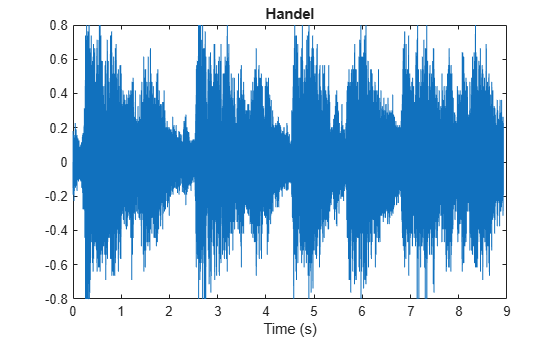# icqt

Inverse constant-Q transform using nonstationary Gabor frames

## Syntax

``xrec = icqt(cfs,g,fshifts)``
``xrec = icqt(___,'SignalType',sigtype)``
``[xrec,gdual] = icqt(___)``

## Description

example

````xrec = icqt(cfs,g,fshifts)` returns the inverse constant-Q transform, `xrec`, of the coefficients `cfs`. `cfs` is a matrix, cell array, or structure array. `g` is the cell array of nonstationary Gabor constant-Q analysis filters used to obtain the coefficients `cfs`. `fshifts` is a vector of frequency bin shifts for the constant-Q bandpass filters in `g`. `icqt` assumes by default that the original signal was real-valued. To indicate the original input signal was complex-valued, use the `'SignalType'` name-value pair. If the input to `cqt` was a single signal, then `xrec` is a vector. If the input to `cqt` was a multichannel signal, then `xrec` is a matrix. `cfs`, `g`, and `fshifts` must be outputs of `cqt`.```
````xrec = icqt(___,'SignalType',sigtype)` designates whether the signal was real-valued or complex-valued. Valid options for `sigtype` are `'real'` or `'complex'`. If unspecified, `sigtype` defaults to `'real'`.```
````[xrec,gdual] = icqt(___)` returns the dual frames of `xrec` as a cell array the same size as `g`. The dual frames are the canonical dual frames derived from the analysis filters.```

## Examples

collapse all

Load and plot the Handel signal.

```load handel t = (0:length(y)-1)/Fs; plot(t,y) title('Handel') xlabel('Time (s)')```Obtain the constant-Q transform of the signal using the `sparse` transform option. Because the transform will be inverted, you must also return the Gabor frames and frequency shifts used in the analysis.

`[cfs,~,g,fshifts] = cqt(y,'SamplingFrequency',Fs,'TransformType','sparse');`

Invert the constant-Q transform and demonstrate perfect reconstruction by showing the maximum absolute reconstruction error and the relative energy error in dB.

```xrec = icqt(cfs,g,fshifts); maxAbsError = max(abs(xrec-y))```
```maxAbsError = 6.9389e-16 ```
`relEnergyError = 20*log10(norm(xrec-y)/norm(y))`
```relEnergyError = -301.4160 ```

## Input Arguments

collapse all

Constant-Q coefficients of a signal or multichannel signal, specified as a matrix, cell array, or structure array. `cfs` must be the output of `cqt`.

Nonstationary Gabor constant-Q analysis filters used to obtain the coefficients `cfs`, specified as a cell array. `cfs` must be the output of `cqt`.

Frequency bin shifts for the constant-Q bandpass filters in `g`, specified as a real-valued vector. `fshifts` must be the output of `cqt`.

Signal type of the original signal, specified as `'real'` or `'complex'`. Use `sigtype` to designate whether the original signal was real-valued or complex-valued. If unspecified, `sigtype` defaults to `'real'`.

## Output Arguments

collapse all

Inverse constant-Q transform, returned as a vector or matrix. If the input to `cqt` was a single signal, then `xrec` is a vector. If the input to `cqt` was a multichannel signal, then `xrec` is a matrix.

Dual frames used in the synthesis of `xrec`, returned as a cell array the same size as `g`. The dual frames are the canonical dual frames derived from the analysis filters.

## Algorithms

The theory of nonstationary Gabor (NSG) frames for frequency-adaptive analysis and efficient algorithms for analysis and synthesis using NSG frames are due to Dörfler, Holighaus, Grill, and Velasco ,. The algorithms used in `cqt` and `icqt` were developed by Dörfler, Holighaus, Grill, and Velasco and are described in ,. In , Schörkhuber, Klapuri, Holighaus, and Dörfler develop and provide algorithms for a phase-corrected CQT transform which matches the CQT coefficients that would be obtained by naïve convolution. The Large Time-Frequency Analysis Toolbox (https://github.com/ltfat) provides an extensive suite of algorithms for nonstationary Gabor frames .

 Holighaus, Nicki, M. Dörfler, G. A. Velasco, and T. Grill. “A Framework for Invertible, Real-Time Constant-Q Transforms.” IEEE Transactions on Audio, Speech, and Language Processing 21, no. 4 (April 2013): 775–85. https://doi.org/10.1109/TASL.2012.2234114.

 Velasco, G. A., N. Holighaus, M. Dörfler, and T. Grill. "Constructing an invertible constant-Q transform with nonstationary Gabor frames." In Proceedings of the 14th International Conference on Digital Audio Effects (DAFx-11). Paris, France: 2011.

 Schörkhuber, C., A. Klapuri, N. Holighaus, and M. Dörfler. "A MATLAB® Toolbox for Efficient Perfect Reconstruction Time-Frequency Transforms with Log-Frequency Resolution." Submitted to the AES 53rd International Conference on Semantic Audio. London, UK: 2014.

 Průša, Z., P. L. Søndergaard, N. Holighaus, C. Wiesmeyr, and P. Balazs. The Large Time-Frequency Analysis Toolbox 2.0. Sound, Music, and Motion, Lecture Notes in Computer Science 2014, pp 419-442.